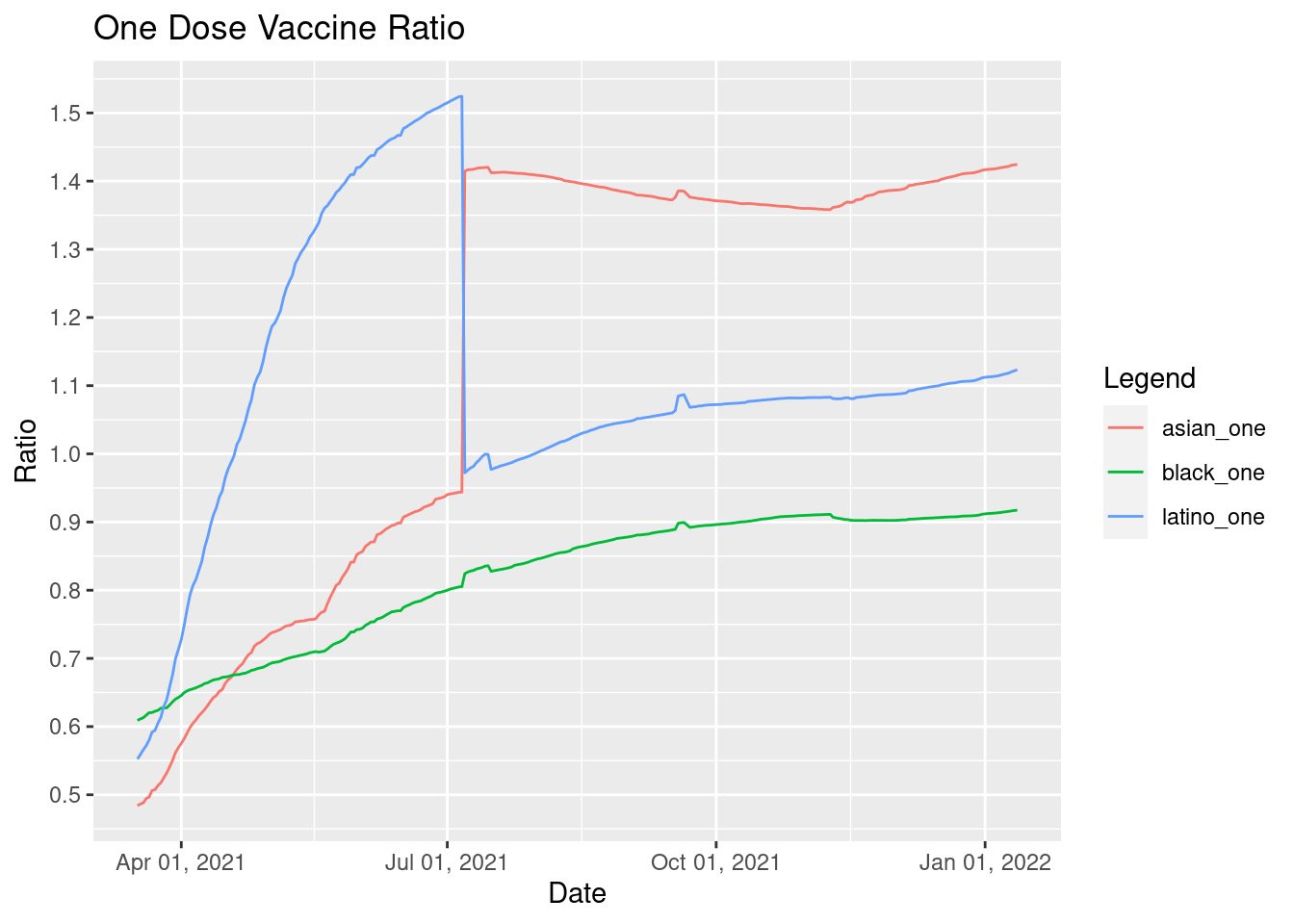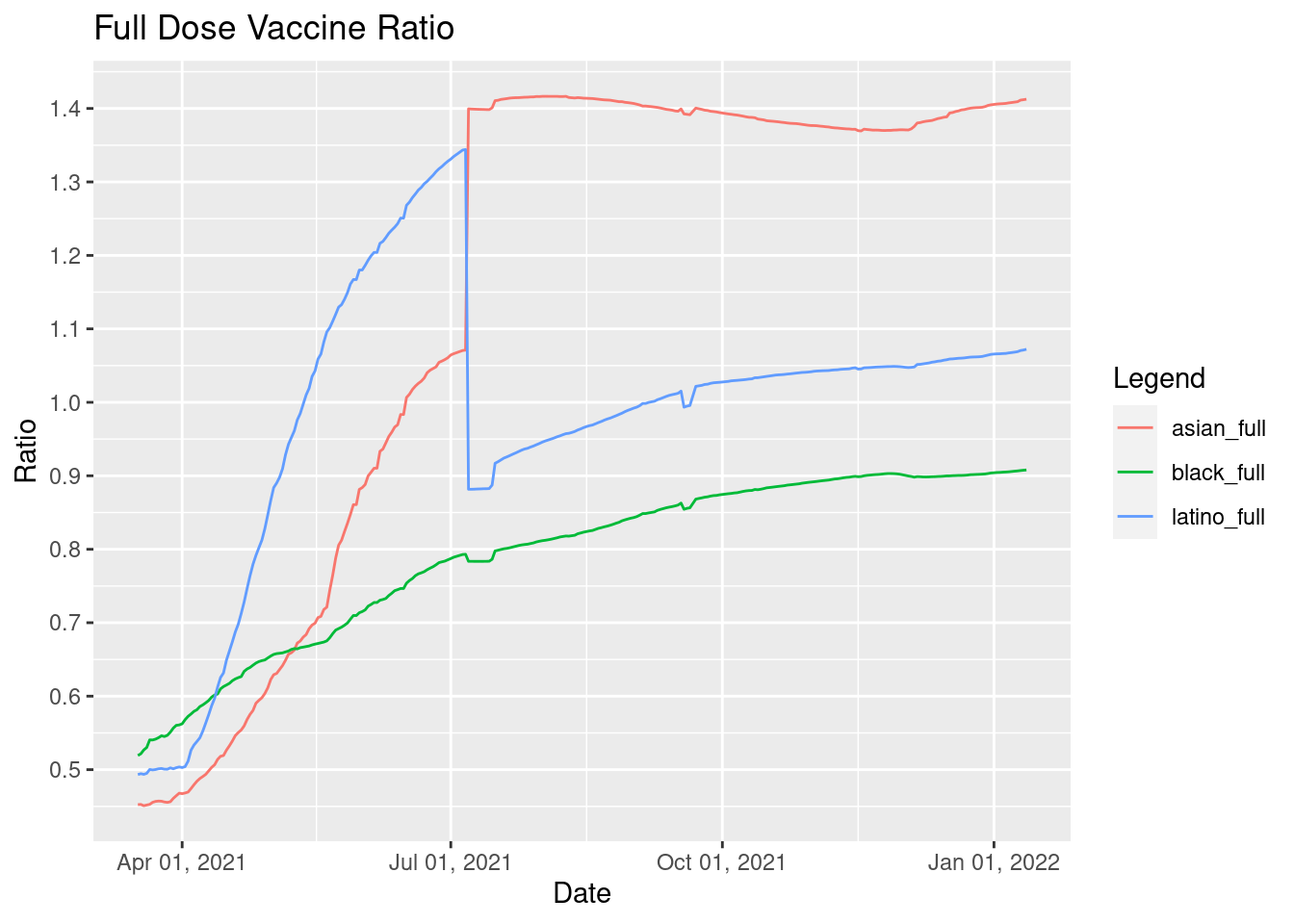Last updated: January 13, 2022

Vaccination data by race/ethnicity in Virginia. As described by Virginia Department of Health (VDH): “Ratios are a comparison to the same rate for the white population. For instance a ratio of 2.0 indicates a rate of twice the white population, while a ratio of 0.5 indicates a rate of half the white population.”

Information has been independently calculated for use on the Metaculus questions linked below. Errors may be present in the results.

What will the cumulative vaccination rate ratio for Black Virginians be in July 2021? What will the cumulative vaccination rate ratio for Hispanic Virginians be in July 2021? What will the cumulative vaccination rate ratio for Asian and Pacific Islander Virginians be in July 2021?

Click the code buttons to see R code used. Source data is from the link below.

library(RSocrata)
library(tidyverse)
library(formattable)
library(reshape)
library(scales)

#define Virginia population statistics, source: https://www.census.gov/quickfacts/VA
population <- 8535519
white_not_latino <- 61.2
black <- 19.9
asian <- 7
latino <- 9.8
white <- 69.4

# this returns output that has for each date the number of vaccinations for each ethnicity and type of vaccination
raw_grouped <- raw_data %>% group_by(report_date,vaccination_status,race_and_ethnicity) %>% summarise(across(people_by_vaccination_status_count, sum))

#convert date column to actual date format
raw_grouped <- raw_grouped %>% mutate(report_date=as.Date(report_date, format = "%m/%d/%Y"))

# filters data to get desired vaccination status and ethnicity
white_one <- raw_grouped %>% filter(vaccination_status == "At Least One Dose",race_and_ethnicity == "White")
black_one <- raw_grouped %>% filter(vaccination_status == "At Least One Dose",race_and_ethnicity == "Black")
latino_one <- raw_grouped %>% filter(vaccination_status == "At Least One Dose",race_and_ethnicity == "Latino")
asian_one <- raw_grouped %>% filter(vaccination_status == "At Least One Dose",race_and_ethnicity == "Asian or Pacific Islander")

white_full <- raw_grouped %>% filter(vaccination_status == "Fully Vaccinated",race_and_ethnicity == "White")
black_full <- raw_grouped %>% filter(vaccination_status == "Fully Vaccinated",race_and_ethnicity == "Black")
latino_full <- raw_grouped %>% filter(vaccination_status == "Fully Vaccinated",race_and_ethnicity == "Latino")
asian_full <- raw_grouped %>% filter(vaccination_status == "Fully Vaccinated",race_and_ethnicity == "Asian or Pacific Islander")

#divide data and add new column and also format by percent
#test_new <- transform(white_one, new = percent(white_one$people_by_vaccination_status_count/8535519,2)) white_one <- transform(white_one, Pop.Percent = white_one$people_by_vaccination_status_count/(population*white_not_latino/100))
Proportion.Black = black_one$people_by_vaccination_status_count/(population*black/100) Proportion.Latino = latino_one$people_by_vaccination_status_count/(population*latino/100)
Proportion.Asian = asian_one$people_by_vaccination_status_count/(population*asian/100) black_one <- transform(black_one, Pop.Percent = percent(Proportion.Black,2), Vaccination.Ratio = Proportion.Black/white_one$Pop.Percent)
latino_one <- transform(latino_one, Pop.Percent = percent(Proportion.Latino,2), Vaccination.Ratio = Proportion.Latino/white_one$Pop.Percent) asian_one <- transform(asian_one, Pop.Percent = percent(Proportion.Asian,2), Vaccination.Ratio = Proportion.Asian/white_one$Pop.Percent)

white_full <- transform(white_full, Pop.Percent = white_full$people_by_vaccination_status_count/(population*white_not_latino/100)) Proportion.Black.Full = black_full$people_by_vaccination_status_count/(population*black/100)
Proportion.Latino.Full = latino_full$people_by_vaccination_status_count/(population*latino/100) Proportion.Asian.Full = asian_full$people_by_vaccination_status_count/(population*asian/100)

black_full <- transform(black_full, Pop.Percent = percent(Proportion.Black.Full,2), Vaccination.Ratio = Proportion.Black.Full/white_full$Pop.Percent) latino_full <- transform(latino_full, Pop.Percent = percent(Proportion.Latino.Full,2), Vaccination.Ratio = Proportion.Latino.Full/white_full$Pop.Percent)
asian_full <- transform(asian_full, Pop.Percent = percent(Proportion.Asian.Full,2), Vaccination.Ratio = Proportion.Asian.Full/white_full\$Pop.Percent)

#melt data so it's all in one table, with labels for what data it's associated with. L1 is a column referencing source.
one_dose_data <- melt(list(black_one = black_one, latino_one = latino_one, asian_one = asian_one), id.vars = "report_date", measure.var = "Vaccination.Ratio")

# below plots the melted data by Report.Data, with melted data of value as y axis. Geom aesthetics group the data by the L1 reference and apply colors.
one_dose_plot <- ggplot(one_dose_data, aes(report_date, value)) +
geom_line(aes(color = L1, group = L1)) +
labs(x="Date", y="Ratio", title="One Dose Vaccine Ratio") +
scale_color_discrete(name="Legend") +
scale_y_continuous(breaks = scales::pretty_breaks(n = 10)) +
scale_x_date(labels = date_format("%b %d, %Y"))

full_dose_data <- melt(list(black_full = black_full, latino_full = latino_full, asian_full = asian_full), id.vars = "report_date", measure.var = "Vaccination.Ratio")

full_dose_plot <- ggplot(full_dose_data, aes(report_date, value)) +
geom_line(aes(color = L1, group = L1)) +
labs(x="Date", y="Ratio", title="Full Dose Vaccine Ratio") +
scale_color_discrete(name="Legend") +
scale_y_continuous(breaks = scales::pretty_breaks(n = 10)) +
scale_x_date(labels = date_format("%b %d, %Y"))

#Ratios Based on One Dose This is what I assume is being used for ratios VDH reports. Numbers are based on ratio of each population to have received one dose.

one_dose_plot#Ratios Based on Full Vaccination I assume this is NOT being used for the ratios VDH reports. Numbers are based on ratio of each population to have been fully vaccinated.

full_dose_plot